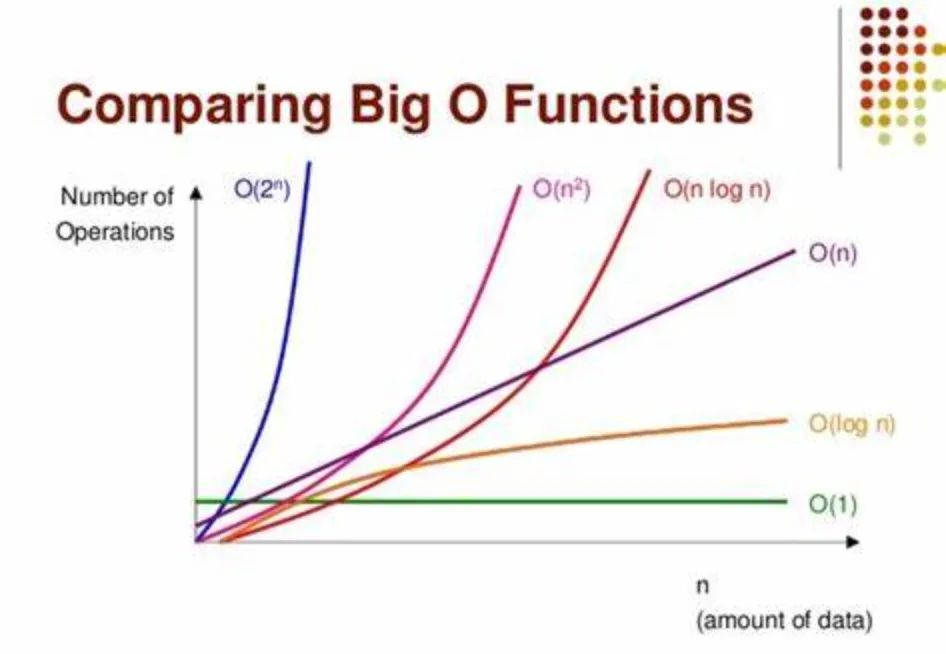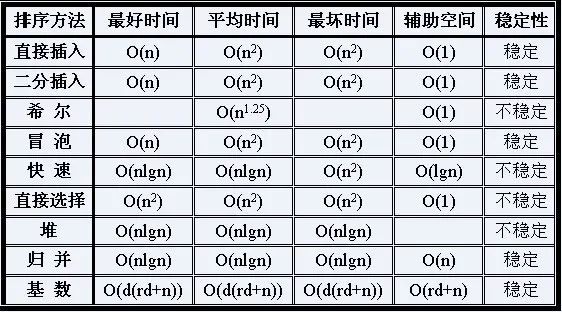# 学习算法必备：时间复杂度与空间复杂度，你了解多少

0收藏

### 前言

• 时间复杂度：用于评估执行程序所消耗的时间，可以估算出程序对处理器的使用程度。
• 空间复杂度：用于评估执行程序所占用的内存空间，可以估算出程序对计算机内存的使用程度。

### 渐进时间复杂度

T(n)=O(f(n))表示存在一个常数C，使得在当n趋于正无穷时总有 T(n) ≤ C * f(n)。简单来说，就是T(n)在n趋于正无穷时最大也就跟f(n)差不多大。也就是说当n趋于正无穷时T(n)的上界是C * f(n)。其虽然对f(n)没有规定，但是一般都是取尽可能简单的函数。### 如何推导时间复杂度

• 找出算法中的基本语句：算法中执行次数最多的语句就是基本语句，通常是最内层循环的循环体。
• 计算基本语句的执行次数的数量级：只需计算基本语句执行次数的数量级，即只要保证函数中的最高次幂正确即可，可以忽略所有低次幂和最高次幂的系数。这样能够简化算法分析，使注意力集中在最重要的一点上：增长率。
• 用大Ο表示算法的时间性能：将基本语句执行次数的数量级放入大Ο记号中。

• 用常数1取代运行时间中的所有加法常数；
• 只保留时间函数中的最高阶项；
• 如果最高阶项存在，则省去最高阶项前面的系数；

### 时间复杂度实例

#### 常数阶O(1)

``````int i = 1;
int j = 2;
int k = 1 + 2;``````

#### 对数阶O(log n)

``````int i = 1; // ①
while (i <= n) {
i = i * 2; // ②
}``````

#### 线性阶O(n)

``````int j = 0; // ①
for (int i = 0; i < n; i++) { // ②
j = i; // ③
j++; // ④
}``````

#### 线性对数阶O(nlogN)

``````    for (int m = 1; m < n; m++) {
int i = 1; // ①
while (i <= n) {
i = i * 2; // ②
}
}``````

#### 平方阶O(n²)

``````int k = 0;
for (int i = 0; i < n; i++) {
for (int j = 0; j < n; j++) {
k++;
}
}``````

``````int k = 0;
for (int i = 0; i < m; i++) {
for (int j = 0; j < n; j++) {
k++;
}
}``````

#### 排序算法对比### 空间复杂度

#### 空间复杂度 O(1)

``````int i = 1;
int j = 2;
int k = 1 + 2;``````

#### 空间复杂度 O(n)

``````int j = 0;
int[] m = new int[n];
for (int i = 1; i <= n; ++i) {
j = i;
j++;
}``````

### 总结一下帖子
视频
声望
粉丝
最近发布
社区精华内容